• ## 游戏抽奖概率模型

千次阅读 2019-04-02 23:34:35
本文主要想罗列出游戏中常见的一些抽奖概率模型，并对其做简要的分析和讨论。 游戏的设计经常会有一些含有随机性质的抽奖设计。比如说卡牌抽奖、购买随机物品等等。它们都可以分为下面这两大类： 1、纯随机概率...
本文主要想罗列出游戏中常见的一些抽奖概率模型，并对其做简要的分析和讨论。

游戏的设计经常会有一些含有随机性质的抽奖设计。比如说卡牌抽奖、购买随机物品等等。它们都可以分为下面这两大类：

1、纯随机概率计算：

纯随机是指：随机结果不受外界影响并且随机的值一直没有变化。比如说大多数购买随机物品的情况。随机物品商店里面获得各种商品的几率都是一样的，不会因为你多次购买不中哪一件商品就会将那间商品的获得概率变大。

2、伪随机概率计算：

伪随机是指：玩家的相关操作或其操作结果会对之后的随机结果造成影响。比如说10 连抽保底的概率模型。

关于纯随机的例子就不多举了，下面说一下我遇到的一些比较典型的伪随机概率模型。

1、10 连抽保底概率模型。

这个模型看《10 连抽保底的概率模型》这篇文章

2、全服根据抽奖人数固定比例数量奖品概率模型1。

假设某件物品A是100个人才能有1个人拥有，并且这个比例必须要得到保证。使用的方法：后端记录总抽奖人数，玩家一抽奖就马上计算出服务器此时总的抽奖次数，如果算上他这次的抽奖，抽奖次数恰好可以被101整除，那么他就是抽中。对于大并发的解决方案就是——中奖、领奖流程与抢数流程分离。用户线程抢数（AtomicInteger），自己判断是否中奖。

3、抽奖不中概率逐渐加大模型。

和模型1（10 连抽保底的概率模型）不一样，这不单单是一个保底的方案。比如说有一类稀有物品：A类物品、普通类型物品：B类物品。保证每抽5次必须是有且仅有1次中A类物品，第1、2、3、4、5次抽中A类概率分别为10%、30%、50%、70%、100%，并且5次之内只要中了A类物品，这五次内后面的几次再中A类物品概率为0。每五次进行一个新的轮回。

4、保证稀有物品最后抽到模型。

如上图所示，玩家只能进行8次抽奖。奖品分为 普通类:普通符文礼盒、金装类:金装符文礼盒、稀有类：圣龙/奥丁英雄。为提高玩家的付费率，策划设计了一种伪随机抽奖方式：

稀有类两种奖品会安排到最后才会被抽取（其中必定有一个是最后一次抽到，另外一个则可能是第6次或第7次）。
金装符文礼包在前四次抽到的几率权重为普通符文礼盒的十分一。
8次抽奖所得组成必须是——4个普通符文礼盒、2个金装符文礼盒、2个稀有类英雄。
下表表示：抽奖次数与所得奖品的权重关系（如果玩家该类奖品已达上限，则权重将改为0）

相关概率模型实现：

对于纯随机概率模型，有很多实现的方案。这里介绍一下MutexRandomUtil，其利用了TreeMap的floorEntry这个方法实现纯随机概率抽取（代码见文末）

对于伪随机概率模型1,3,4这种抽过即无法再中第二次的情况，借鉴MutexRandomUtil，我的实现是将所有将会抽到的物品及其抽取概率值都封装到一个类中。每次从该类随机抽取物品，抽到的物品都进行移除，这样既可以保证概率的延续性，也能保证抽中奖品不重复。（代码见文末）

public class MutexRandomUtil<T> {
private TreeMap<Double, T> map = new TreeMap<Double, T>();
private double endRate = 0;

public MutexRandomUtil() {
}

public void registerData(double rate, T t) {
if (rate <= 0) {
return;
}
map.put(endRate, t);
endRate += rate;
}

public T random() {
double r = RandomUtil.avgRandom(0, endRate);
Map.Entry<Double, T> e = map.floorEntry(r);
return e != null ? e.getValue() : null;
}
}

public class MutexRemoveRandom<T> {
private TreeMap<Double, Entry<T>> map = new TreeMap<Double, Entry<T>>();
private double endRate = 0;
private static Random r = new Random();

public void registerData(double rate, T t) {
if (rate <= 0)
return;
map.put(endRate, new Entry<T>(t, rate));
endRate += rate;
}

public T randomAndRemove() {
double r = avgRandom(0, endRate);
Double key = map.floorKey(r);
if (key != null) {
T t = map.get(key).t;
reorganizeMap(key);
return t;
} else {
return null;
}
}

private void reorganizeMap(Double key) {
TreeMap<Double, Entry<T>> tempMap = new TreeMap<>();
map.remove(key);
double tempEndRate = 0;
for (Map.Entry<Double, Entry<T>> entry : map.entrySet()) {
tempMap.put(tempEndRate, entry.getValue());
tempEndRate += entry.getValue().rate;
}
this.endRate = tempEndRate;
this.map = tempMap;
}

public static double avgRandom(double min, double max) {
if (min > max) {
double temp = max;
max = min;
min = temp;
}
double rNum = r.nextDouble() * (max - min);
return rNum + min;
}

private static class Entry<T> {
final T t;
final double rate;

Entry(T t, double rate) {
this.t = t;
this.rate = rate;
}
}
}


展开全文• ## 游戏抽卡概率模型

千次阅读 多人点赞 2020-11-22 13:36:29
游戏抽卡概率模型 目前的中国游戏市场，最热门的莫过于免费抽卡手游。游戏厂商为了盈利，设计出有不同规则的卡池，让玩家抽卡获取稀有的人物和武器。这一机制吸引了很多玩家，首先因为手游是免费的，入手门槛较低，...
目前的中国游戏市场，最热门的莫过于免费抽卡手游。游戏厂商为了盈利，设计出有不同规则的卡池，让玩家抽卡获取稀有的人物和武器。这一机制吸引了很多玩家，首先因为手游是免费的，入手门槛较低，其次很多人喜欢抽卡获取角色和武器的成就感。为了更吸引玩家，游戏策划就得设计出能够让大部分玩家，无论是欧皇还是非酋满意的抽卡机制。本文将简要分析目前游戏厂商可能使用的抽卡机制（仅属于个人猜想加一些网上的资料）。
文章目录抽卡机制的大致分类伪随机模型十连保底概率增加模型参考资料源代码
抽卡机制的大致分类

最简单的抽卡模型就是每次抽卡的概率都是固定的，也就是说每次抽卡的概率都是$p$,我们可以称之为纯随机。那么玩家第$n$次抽中的随机变量分布就是一个几何分布。这种抽卡模型很明显对于非酋非常不友好，理论上可能存在抽了很多很多次仍然没有抽中的人存在。所以纯随机模型在抽卡游戏中是不会使用的。
对应于纯随机的，我们可以称之为伪随机（注意这里的伪随机和计算机随机数概念中的伪随机不是一个东西）。伪随机就是说每次抽卡的概率都是是会进行改变的。下面就重点介绍几种可能的伪随机模型

伪随机模型

十连保底
十连保底应该是我们在游戏中最常见到的概率模型。如果连抽十次，前九次都没有抽中的话，那么第十次就必定会抽中。这种概率模型会导致很多玩家都是依靠十连保底，并且概率质量函数图像会在第十次突然上升，显得不均匀。
例如，如果我们设定抽到A卡的概率为0.1，那么我们得出的该概率质量函数和累积分布函数的图像就是下图。可以算出数学期望为6.5，并且大约60%的玩家在前9次抽到，而所谓的非酋也就是触发保底的玩家占到了40%。如果我们用程序模拟10000次在这种概率模型下的抽卡，我们可以得出下面的分布。从图中可以看出，大部分玩家抽100次会得到16-20个想要得到的。这种十连保底的模型一般用于四星角色或者武器。四星虽然没有五星稀有，但是也是需要一定的保障以确保玩家甚至连四星都抽不到。

概率增加模型
那么对于更加稀有的五星角色和武器来说，十连保底肯定就显得太过容易了。这个时候策划一般希望玩家差不多抽几十发可以抽中一个，所以就需要比如100抽保底这样的机制。但是如果这个时候继续采用以上的前面的概率都不变，那么很容易想到这样子的话大部分玩家都会是100发保底…所以就需要调整该概率。
首先，我们设定一个初始概率$p$，然后如果没有抽中，那么下一次抽卡概率就给他一个增量$\alpha$，如果抽中，那么就回归初始概率。所以，第X次抽中的概率就是$p(x)=p+\alpha\times (x-1)$，概率质量函数
$P(X)=\Pi^{x-1}_{i=1}\lbrack(1-p(i))\rbrack\times p(x)=\Pi^{x-1}_{i=1}[(1-p-\alpha\times(i-1))]\times(p+\alpha\times(x-1))$
我们设定$p=0.01, \alpha=0.01$，然后画出图像之后发现，这个图像居然很像是一个正态分布的图像，所以顺便把正态分布的图像画了出来对比，虽然也不知道为什么这两张图这么像。在这个概率模型下，由于100次的时候$p(100)=0.01+99\times0.1=1$，所以实现了一个100抽保底的效果。但是策划希望的是100抽保底，并且大部分人应该是在五六十抽的时候抽到的，而在这个模型中，超过90%的人在前二十抽就抽到了，这显然不符合预期。所以在这个模型的基础上，我们需要进行相应的调整，让模型的最高点向右偏移。我们由此可以使用一下模型：前50次抽卡概率固定，后50次抽卡使用上面的模型，每次抽卡概率增加。这样我们得出的该概率质量函数就是
$p(x)= \begin{cases} p, & x<50 \\ p+\alpha(x-50), &x>=50 \end{cases}$
和上面一样，如果需要满足100抽保底的话，让$p=0.02,\alpha=0.02$。其概率质量函数和累积分布函数图像如下：这张图我认为说可以用作五星的概率模型了。70发之前大部分人都能够抽到，并且集中在40发到70发，占了43.69%。这也可以视作游戏玩家口中的“低保”。
我们使用上面的模型模拟10000次100连抽，得到的结果如下：这个结果个人认为就非常像真实游戏中的结果了，大部分人都只能够抽到1-2发，并且很多人都是依靠低保的（这很真实）

最后还写了一个模拟原神中抽卡的程序，并没有使用概率增加，仅仅只是用保底的跳跃性的概率变化来实现保底的功能，以后可能在优化一下。这是一次100抽的结果四星角色三次触发十连保底，五星角色一次在43抽抽中，一次在47次抽中，一共抽到了2个五星，9个四星。感觉这个概率貌似比真实中更高一些，以后再优化优化…
参考资料
明日方舟抽卡概率和保底机制研
游戏抽奖概率模型
源代码
概率模型GitHub
原神模拟GitHub


展开全文python
• 机器学习常见概率模型概率模型Bernoulli 分布Multinoulli 分布高斯分布指数分布和Laplace 分布分布的混合 概率模型 Bernoulli 分布 Bernoulli 分布（Bernoulli distribution）是单个二值随机变量的分布。它由单个...
机器学习常见概率模型概率模型Bernoulli 分布Multinoulli 分布高斯分布指数分布和Laplace 分布分布的混合
概率模型
Bernoulli 分布
Bernoulli 分布（Bernoulli distribution）是单个二值随机变量的分布。它由单个参数ϕ 控制，ϕ 给出了随机变量等于1 的概率。它具有如下的一些性质：Multinoulli 分布
Multinoulli 分布（multinoulli distribution）或者范畴分布（categorical distribution）是指在具有k 个不同状态的单个离散型随机变量上的分布，其中k 是一个有限值，范畴分布范畴分布实际是对二维泊松分布在维数上的扩展。
高斯分布
实数上最常用的分布就是正态分布（normal distribution），也称为高斯分布（Gaussian distribution），公式和分布如下：采用正态分布在很多应用中都是一个明智的选择。当我们由于缺乏关于某个实数上分布的先验知识而不知道该选择怎样的形式时，正态分布是默认的比较好的选择，其中有两个原因。
第一，我们想要建模的很多分布的真实情况是比较接近正态分布的。中心极限定理（central limit theorem）说明很多独立随机变量的和近似服从正态分布。这意味着在实际中，很多复杂系统都可以被成功地建模成正态分布的噪声，即使系统可以被分解成一些更结构化的部分。
第二，在具有相同方差的所有可能的概率分布中，正态分布在实数上具有最大的不确定性。因此，我们可以认为正态分布是对模型加入的先验知识量最少的分布。
指数分布和Laplace 分布
在深度学习中，我们经常会需要一个在x = 0 点处取得边界点(sharp point) 的分布。为了实现这一目的，我们可以使用指数分布（exponential distribution）：一个联系紧密的概率分布是Laplace 分布（Laplace distribution），它允许我们在任意一点 $\mu$ 处设置概率质量的峰值。分布的混合
通过组合一些简单的概率分布来定义新的概率分布也是很常见的。一种通用的组合方法是构造混合分布（mixture distribution）。混合分布由一些组件(component)分布构成。
Reference: LeCun, Yann, Yoshua Bengio, and Geoffrey Hinton. “Deep learning.” nature 521.7553 (2015): 436.


展开全文• 1、概率模型 机器学习中的很多模型可以根据概率分布形式分为生成模型和判别模型，其中生成模型以输入输出的联合分布P(X,Y)为基础建模，如朴素贝叶斯、隐马尔可夫模型；判别模型以条件概率分布P(Y|X)为基础建模，如...
1、概率模型
机器学习中的很多模型可以根据概率分布形式分为生成模型和判别模型，其中生成模型以输入输出的联合分布P(X,Y)为基础建模，如朴素贝叶斯、隐马尔可夫模型；判别模型以条件概率分布P(Y|X)为基础建模，如最大熵模型、条件随机场等。这几个模型之间有一定的关系，它们的关系如下：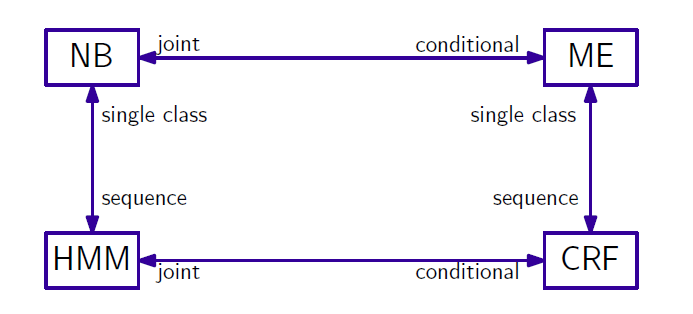其中，NB表示朴素贝叶斯，ME表示最大熵，HMM表示隐马尔科夫，CRF表示条件随机场。joint联合分布，conditional条件分布。single class输出单一类别，sequence输出序列。例如，朴素贝叶斯将输出y扩展成序列(y1,y2,...,yn)$(y_1,y_2,...,y_n)$，就可以以此为基础构造HMM；在满足输入条件下的HMM可以扩展成CRF。

这里面，朴素贝叶斯假设最强 ，因为它要求所有输入特征之间条件独立，如P(y|x1,x2,...,xn)=∏i=1nP(y|xi)$P(y|x_1,x_2,...,x_n)=\prod\limits_{i=1}^nP(y|x_i)$；这是一种为计算方便而做的近似假设，然而现实中基本不会有模型符合输入特征间的独立，因此以朴素贝叶斯建模一般会有精度损失。
隐马尔科夫模型进了一步，它考虑一定的变量相关性，如马尔科夫假设状态序列中，当前状态只与其前一个状态有关，如：
P(X,Y)=∏i=0nP(yi|yi−1P(xi|yi)$P(X,Y)=\prod\limits_{i=0}^nP(y_i|y_{i-1}P(x_i|y_i)$
但是HMM只考虑了状态之间的邻接关系，没有考虑观测序列间的关系，条件随机场刚好弥补了这个缺陷。所以条件随机场是一个相对比较完善的模型，但代价是计算复杂性的提高。

2、概率图模型
上面讲到的概率模型可以用图的形式表示出来，称为概率图模型。概率图模型用图中结点表示随机变量，结点之间的边表示变量间的概率相关关系。

在概率图中，两结点没有边相连，说明两节点是条件独立的，比如P(a,b|c)=P(a|c)⋅P(b|c)$P(a,b|c)=P(a|c)\cdot P(b|c)$。在概率图中，结点间全连接是不包含任何概率分布信息的，所以我们更关注的是哪些边是缺失的，这些缺失的边表示边连接的结点条件独立。

下图中的两个图是概率图的两种表示形式，一个是独立图，一个是因子图。通过条件独立的条件，可以将一个复杂的概率分布分解成简单的概率分布乘积，如下图中（a）,联合概率分布P(x1,x2,y)=P(x1)⋅P(x2)⋅P(y|x1,x2)$P(x_1,x_2,y)=P(x_1)\cdot P(x_2)\cdot P(y|x_1,x_2)$。
若定义因子，也称势函数Ψs$\Psi_s$为概率分布的分解因子，对任意概率图G=（V,E）,有：

p(V)=∏sΨs(Vs)$p(V)=\prod\limits_s\Psi_s(V_s)$

其中，s表示随机变量构成的集合，Vs$V_s$表示该集合中包含的变量。
则可以将P(x1,x2,y)$P(x_1,x_2,y)$写成P(x1,x2,y)=Ψ1⋅Ψ2⋅Ψ3$P(x_1,x_2,y)=\Psi_1\cdot \Psi_2\cdot \Psi_3$，这里的Ψi$\Psi_i$分别与独立图中的概率对应。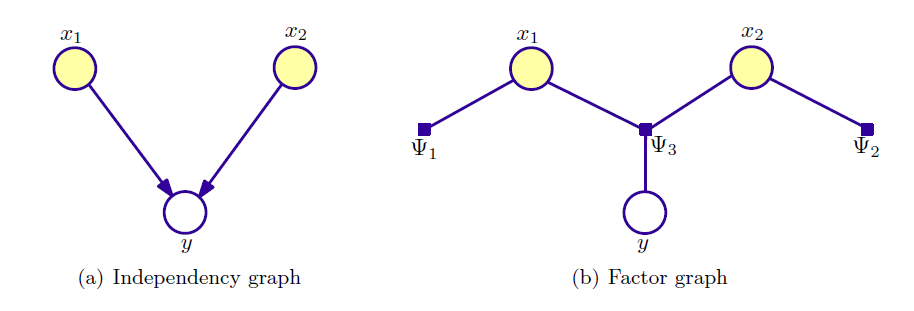概率图模型可大致分为两类：一类是有向图模型，表示变量间的依赖关系，也称为贝叶斯网；一类是无向图模型，表示变量间的相关关系，也称为马尔科夫网或马尔科夫随机场。
2.1 有向图模型
在有向图中，边表示了变量之间的一种依赖关系。联合分布概率可以写作是所有变量在在父节点条件下的概率乘积：

P(V)=∏i=1KP(vk|vnk)$P(V)=\prod\limits_{i=1}^KP(v_k|v_k^n)$

如下图所示的隐马尔可夫有向图，联合概率可以写作：

P(x1,x2,x3,y1,y2,y3)=Ψ1(y1)⋅Ψ2(x1,y1)⋅Ψ3(x2,y2)⋅Ψ4(x3,y3)⋅Ψ5(y1,y2)⋅Ψ6(y2,y3)$P(x_1,x_2,x_3,y_1,y_2,y_3)=\Psi_1(y_1)\cdot \Psi_2(x_1,y_1)\cdot \Psi_3(x_2,y_2)\cdot \Psi_4(x_3,y_3)\cdot \Psi_5(y_1,y_2)\cdot \Psi_6(y_2,y_3)$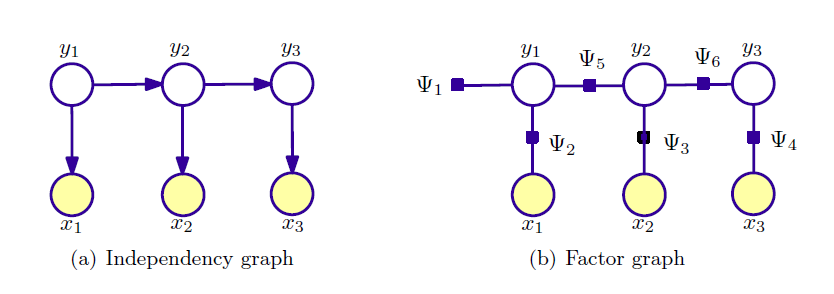2.2 无向图模型
在无向图模型中，有个团和最大团的概念，表示了变量之间的关系。团的意思是一些随机变量结点构成的子集中，两两结点都有边相连，如下图中(1,2)、（1,2,5）等；最大团表示结点构成的团中再添加任何一个新结点后都不会构成团，如（1,4,5）。在一些线性链结构的无向图，如线性链条件随机场中，最大团只考虑(yj−1,yj,x$y_{j-1},y_j,x$)。像有向图的分解一样，无向图也可以分解，无向图是基于最大团进行分解，如下：
P(V)=1Z∏C∈CΨC(VC)$P(V)=\frac 1 Z\prod\limits_{C\in \mathcal{C}}\Psi_C(V_C)$
其中每个最大团对应一个势函数ΨC$\Psi_C$。是不是跟最大熵模型的形式很相似？因为最大熵模型也是一个无向图模型。像在最大熵模型中一样，Z是一个归一化因子，如下：
Z=∑V∏C∈CΨC(VC)$Z=\sum\limits_V\prod\limits_{C\in \mathcal{C}}\Psi_C(V_C)$
一般，势函数要求严格非负，所以在使用中会选择指数函数作为势函数。如下图的一个最大熵模型，可以写作：
P(y|x)=1Zλ(x)eλ1f1⋅eλ2f2⋅eλ3f3$P(y|x)=\frac 1{Z_{\lambda}(x)}e^{\lambda_1f_1}\cdot e^{\lambda_2f_2}\cdot e^{\lambda_3f_3}$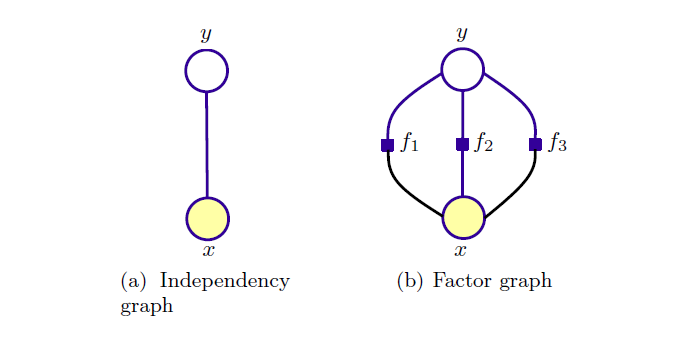有向图与无向图的一个主要区别在于概率分布的分解不同，在概率有向图中，分解因子是条件概率分布；在无向图中，分解因子可以是任意函数，无向图不需要说明变量间是如何关联的，而是将在一个团中的变量作为一个整体来看。
**3、条件随机场**
在前面，我们说可以把隐马尔科夫模型看作是对贝叶斯模型的序列化；类似地，我们可以把条件随机场看作是对最大熵模型的序列化。条件随机场并不要求线性序列，即它可以是任意结构的，通常我们使用较多的是线性链随机场，它可以看作是有条件的HMM(即加入了观测序列x的条件)。
条件随机场属于判别模型，即它要求出在观测序列x的条件下得到可能输出序列y的概率P(y|x)$P(y|x)$。
由上面的无向图分解公式
P(V)=1Z∏C∈CΨC(VC)$P(V)=\frac 1 Z\prod\limits_{C\in \mathcal{C}}\Psi_C(V_C)$
条件概率P(y|x)$P(y|x)$可以写作：

p(y|x)=p(x,y)p(x)$p(y|x)=\frac{p(x,y)}{p(x)}$
=p(x,y)∑y′p(y′,x)$\qquad\qquad=\frac {p(x,y)}{\sum_{y^{'}}p(y^{'},x)}$
=1Z∏C∈CΨC(xC,yC)1Z∑y′∏C∈CΨC(xC,yC)$\qquad\qquad\qquad\quad=\frac{\frac 1{Z}\prod_{C\in\mathcal{C}}\Psi_C(x_C,y_C)}{\frac 1{Z}\sum_{y^{'}}\prod_{C\in\mathcal{C}}\Psi_C(x_C,y_C)}$
=1Z(x)∏C∈CΨC(xC,yC)$\qquad\qquad\qquad\qquad\quad=\frac 1{Z(x)}\prod_{C\in\mathcal{C}}\Psi_C(x_C,y_C)$

其中，

Z(x)=∑y′∏C∈CΨC(xC,yC)$Z(x)=\sum_{y^{'}}\prod_{C\in\mathcal{C}}\Psi_C(x_C,y_C)$

下面介绍一下常用的线性链条件随机场，
线性链CRFs是条件随机场中的一种特殊结构，与隐马尔科夫一样，输出形成一个线性序列，如下图：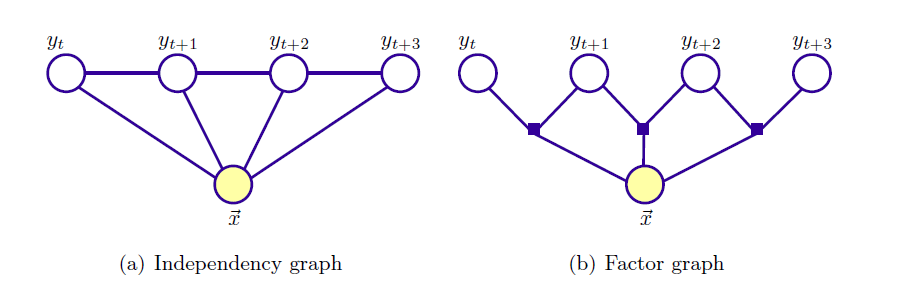根据上面的公式，其条件概率可以写作，

p(y|x)=1Z(x)∏j=1nΨj(x,y)$p(y|x)=\frac 1{Z(x)}\prod\limits_{j=1}^n\Psi_j(x,y)$

其中，n+1表示输出状态序列长度，n为势函数个数。
由图可知，状态yj$y_j$与输入x$x$和yj−1$y_{j-1}$有关，特征函数可以写作：
f(yj−1,yj,x,j)$f(y_{j-1},y_j,x,j)$
势函数：
Ψj(x,y)=exp(∑i=1mλifi(yj−1,yj,x,j))$\Psi_j(x,y)=exp\left(\sum\limits_{i=1}^m\lambda_if_i(y_{j-1},y_j,x,j)\right)$
进而，线性链CRFs的条件概率分布可以写作，

pλ(y|x)=1Zλ(x)exp(∑nj=1∑i=1mλifi(yj−1,yj,x,j))$p_{\lambda}(y|x)=\frac 1{Z_{\lambda}(x)}exp\left(\sum_{j=1}^n\sum\limits_{i=1}^m\lambda_if_i(y_{j-1},y_j,x,j)\right)$

其中，Zλ(x)$Z_{\lambda}(x)$是归一化因子，

Zλ(x)=∑y∈Yexp(∑nj=1∑i=1mλifi(yj−1,yj,x,j))$Z_{\lambda}(x)=\sum\limits_{y\in \mathcal{Y}}exp\left(\sum_{j=1}^n\sum\limits_{i=1}^m\lambda_if_i(y_{j-1},y_j,x,j)\right)$

展开全文条件随机场 隐马尔科夫
• ## 信息检索概率模型

千次阅读 2014-03-19 13:25:42
基本的概率模型（简单概率模型）：  将文档分为两个部分：第一个是和查询语句相关的，第二个和查询非相关的 与查询语句相关的P(D|R) document related  与查询语句不相关的P(D|NR) document unrelated  代码...搜索 数据库 idf
•  出于兴趣，在VC编译环境下，通过C++语言，模拟实现了高尔顿钉板的概率模型。本程序中定义了两个类，Ball和Holder类，Ball 是定义的小球类，Holder是定义的钉板类。Holder类主要负责画图显现模板框架，以及当C++
• 什么是结构化概率模型 "结构化概率模型"（structured probabilistic model），是一类用图形模式表达基于概率相关关系的模型的总称，也称“图模型”（graphical model）英文简称，PGM  概率图模型具有...
• 基于概率模型的聚类 我们之所以在数据集上进行聚类分析，是因为我们假定数据集中的对象属于不同的固有类别。即聚类分析的目的就是发现隐藏的类别。 从统计学上讲，我们可以假定隐藏的类别是数据空间的一个分布，可以...聚类学习
• PRISM— probabilistic model checker 概率模型检测器 骰子模型 The dieexample http://www.prismmodelchecker.org/tutorial/die.php  与马尔科夫链有关 PRISM code： dtmc   module diePRISM 马尔科夫链
• ## 贝叶斯概率模型

千次阅读 2020-07-18 13:18:02
一、贝叶斯模型 思想 贝叶斯理论的思想，简单点讲就是要在已知条件的前提下，先设定一个假设，然后通过先验实验来更新这个概率。 工作流程 优点 1. 基于古典数学理论，分类效率稳定 2. 对缺失数据不敏感 3. 准确...机器学习
• 基本概率模型这里简单介绍三个概念，古典概型，频率学派，贝叶斯学派。 古典概型 这个模型下，随机实验所有可能的结果是有限的，并且每个基本结果发生的概率是相同的 比如：投掷一枚均匀硬币，结果只有两种（假设...
• 二值因变量模型 在统计学中，有一种离散变量为二值变量(又称虚拟变量、哑变量)...探讨Logistic模型之前，我们先从线性概率模型(Linear Probability Model，LPM)谈起，然后逐步介绍Logistics模型以及其他非线性模型.统计学 数据挖掘
• 一、Matlab相关的概率命令： ...二、概率公式：全概率模型、贝叶斯公式 分布：1、正态分布——如果决定实验结果X是大量随机因素的总和，假设各个因素之间近似独立，并且每个...数学建模
• CRF和MRF概率模型的关系最近再次阅读了文献Discriminative fields for modeling spatial dependencies in natural images，对CRF模型和MRF模型有了进一步的理解。总体来说这两者都是属于概率图模型范畴，MRF的理论是...CRF 区别
• ## 结构化概率模型

千次阅读 2018-12-09 21:00:41
机器学习的算法经常会涉及到在非常多的随机变量上的概率分布。通常，这些概率分布涉及到的直接相互作用都是介于非常少的变量之间的。使用单个函数来描述整个联合概率分布是非常低效的 (无论是计算上还是统计上)。 ...机器学习 概率分布
• discriminative model 和 generative model是机器学习算法中两种概率模型，用来实现对训练样本的概率分布进行建模，在实践中由于经常混淆，现在通过查阅资料，将两者的分别总结于此。 不妨用stackoverflow上的一段...机器学习
• 概率模型与计算机视觉 林达华 美国麻省理工学院（MIT）博士 上世纪60年代, Marvin Minsky 在MIT让他的本科学生 Gerald Jay Sussman用一个暑假的时间完成一个有趣的Project : “link a camera to a compu计算机视觉
• 温故知新 1.抽样模型   2.放回抽样模型   3.盒子模型    4.生日问题    5.会面问题   7. 蒲丰投针      8.配对问题    9.摸彩问题
• 9月开始，本博客开始focus到Topic Modeling及LDA概率模型，研读机器学习的经典著作PRML，这本书还没有中文翻译版，只能啃英文原版了，也好，原版质量更高 将陆续推出Topic Modeling及LDA概率模型，PRML研读系列文章...
• 生成概率模型（Generative Model） 我们还是从分类问题说起： 当我们把问题问题看做是一个回归问题， 分类是class 1 的时候结果是1 分类为class 2的时候结果是-1； 测试的时候，结果接近1的是class1 ，结果...
• 新一代AI顾泽苍：自组织概率模型SDL主导的机器学习(公号回复“顾泽苍AI”下载PDF资料，欢迎转发、赞赏支持科普) 原创： 秦陇纪 科学Sciences 今天 科学Sciences导读：自组织概率模型SDL主导的机器学习是新一代人工...
• 本文介绍GMM算法，也就是高斯混合模型，或则更准确的说，应该称为高斯线性混合模型。 高斯分布 又称为正太分布，常态分布，是自然界大量存在的、最为常见的分布。例如人类和动物的身高，体重，测量误差...
• 此文章解释指数分布族，广义重点内容线性模型，以及softmax回归的概率解释推导。1.指数分布族指数分布族即为指数形式的概率分布：其中，T(y)为充分统计量，tau为自然参数。大多数的概率分布都可以转化为指数分布模型...机器学习 指数分布族 广义线性模型
• 高斯分布，也就是所谓的正态分布，在1维的情况下，参数是均值和方差，比较好理解的。在高维的情况下，输入x变成了一个n维的向量，相应高维高斯分布的参数也就变成了...而对于由K个单高斯分布组成的高斯混合模型，由...基础知识
• 1、 概率语言模型一句话的概率公式：根据一句话中的前文预测下一个词：根据链式概率法则：根据马尔科夫假设，当前词只和前n个词有关系：2. 大名鼎鼎的 n-gram模型模型想要预测的下一个词，为句子中的历史词语。...NLP 机器学习 神经网络 词向量
• 本文讨论局部概率模（Structured-CPDs）。探索网络的局部结构（CPDs），理清条件概率分布中父节点变量间的关系，会发现更多好的独立性质，以此稀疏化网络的表示。同时对于连续变量，也避免了全概率分布表难以表达...
• 肤色高斯概率模型，主要将颜色空间从RGB转换为YCrCb，计算肤色的相似度，确定根据概率阈值筛选出肤色区域。但高斯概率模型中肤色均值及协方差矩阵需要提前确定，并且肤色均值对检测结果影响非常大，需要根据实际情况...人脸检测
• 简介 非监督机器学习（Unsupervised Machine Learning）中的数据分布密度估计（Density Estimation）、样本采样（Sampling）与生成...完成这类任务的模型称为生成式模型（Generative Model）。其实，另一...机器学习 生成式模型 概率密度估计
• 2 非概率模型 VS 概率模型 2.1 非概率模型概率模型指的是直接学习输入空间到输出空间的映射h，学习的过程中基本不涉及概率密度的估计，概率密度的积分等操作，问题的关键在于最优化问题的求解。通常，为了学习...机器学习 统计学习 监督学习 数据挖掘
• 此文章解释指数分布族，广义重点内容线性模型，以及逻辑回归的概率解释推导。1.指数分布族指数分布族即为指数形式的概率分布：其中，T(y)为充分统计量，tau为自然参数。大多数的概率分布都可以转化为指数分布模型，...机器学习 指数分布族 广义线性模型...